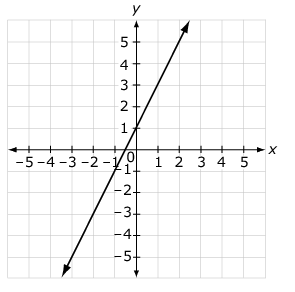# Expressions and Equations / Prop. Relationships to Linear Equations

Activity 14 of 18
In this lesson students use previous experience with proportional relationships of the form $$y = kx$$ to consider relationships of the form $$y = mx$$ and eventually $$y = mx+b$$.

## Planning and Resources

Objective
Students should be able to connect the unit rate in a proportional relationship to the slope of its graph using similar triangles, and connect the equation for a proportional relationship, $$y = mx$$, to the equation for a line, $$y = mx+b$$.

Vocabulary
proportional relationship
linear equation
intercept

Standard:

## Content Group - Lorem

#### Understanding

This lesson helps students to use the point slope form of a line to move from graphs of proportional relationships to graph of any line.

### What to look for

Student discussion may provide an opportunity to discuss why the denominator of a fraction cannot be zero.

### Sample Assessment

Consider the graph of a line.Which equation has a slope greater than the slope for the line shown?

a. $$y = 3x-1$$      b. $$y = \frac{x}{2}+4$$

c. $$y = 2x+2$$      d. $$y = \frac{x}{3}-3$$